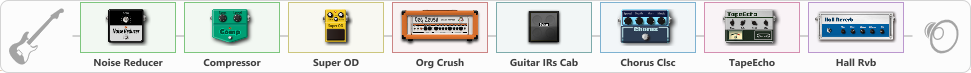Discussion in 'ToneLib-GFX presets' started by truss van halen, Feb 21, 2022.

1. ### truss van halenWell-Known Member

Preset name: I was made for loving you - KISS

Effects chain:Effect: "Noise Reducer" (Dynamics / Filter), active - "yes"
{
"Sens" = 100
"Mode" = Hard
}

Effect: "Compressor" (Dynamics / Filter), active - "yes"
{
"Sense" = 32
"Level" = 43
}

Effect: "Super OD" (Overdrive / Distortion), active - "yes"
{
"Drive" = 0
"Tone" = 67
"Level" = 61
}

Effect: "Org Crush" (Amp simulators), active - "yes"
{
"Gain" = 17
"Bass" = 70
"Middle" = 85
"Treble" = 42
"Presence" = 55
"Master" = 64
"Level (dB)" = 4
}

Effect: "Guitar IRs Cab" (Cabinets), active - "yes"
{
"Model" = Marshall 1960A (4x12")
"Mic Position" = Middle
"Mic Distance" = Near
"Low Cut (Hz)" = 60
"Hi Cut (kHz)" = 20.0
"Mix" = 100
"Level (dB)" = 1
}

Effect: "Chorus Clsc" (Modulation / Sfx), active - "yes"
{
"Speed" = 4.0
"Depth" = 26
"Center" = 2.8
"Mode" = Stereo
}

Effect: "TapeEcho" (Delay), active - "yes"
{
"Time" = 442
"Feedback" = 34
"Tone" = 65
"LoDamp" = 19
"Mix" = 65
}

Effect: "Hall Rvb" (Reverberation), active - "yes"
{
"Time" = 6.3
"PreDelay" = 0
"LoDamp" = 0
"HiDamp" = 17
"Mix" = 57
}

Note: You will need to download and install the ToneLib-GFX software to use the preset.